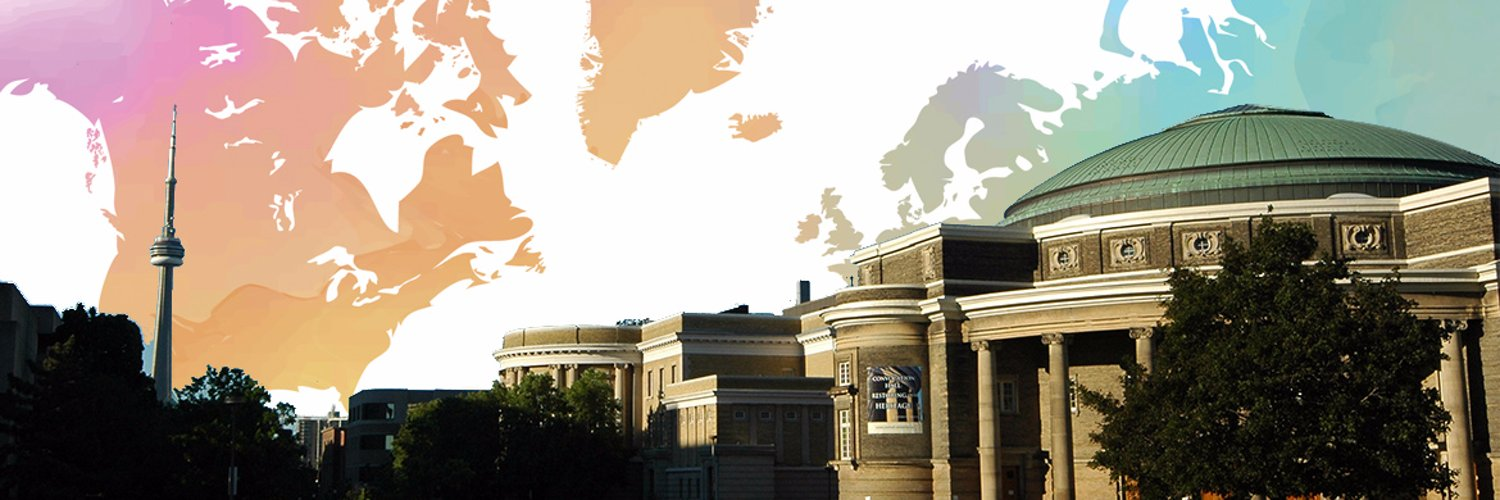# John ScherkAssociate Professor | University of Toronto, Department of Mathematics

Toronto, ON, CANADA

Dr. Scherk's research interests lie in algebraic geometry.

## Biography

Dr. Scherk's research interests lie in algebraic geometry, more specifically in singularities, Hodge theory, and compactifications of locally symmetric spaces.

## Industry Applications (2)

Education/Learning Research

## Research Interests (1)

Algebraic Geometry

## Articles (3)

The fundamental group of reductive Borel-Serre and Satake compactifications Asian Journal of Mathematics

2015-07-10

2011

Let G be an almost simple, simply connected algebraic group defined over a number field k, and let S be a finite set of places of k including all infinite places. Let X be the product over v∈S of the symmetric spaces associated to G(kv), when v is an infinite place, and the Bruhat-Tits buildings associated to G(kv), when v is a finite place. The main result of this paper is an explicit computation of the fundamental group of the reductive Borel-Serre compactification of Γ∖X, where Γ is an S-arithmetic subgroup of G. In the case that Γ is neat, we show that this fundamental group is isomorphic to Γ/EΓ, where EΓ is the subgroup generated by the elements of Γ belonging to unipotent radicals of k-parabolic subgroups. Analogous computations of the fundamental group of the Satake compactifications are made. It is noteworthy that calculations of the congruence subgroup kernel C(S,G) yield similar results...

The ramification polygon for curves over a finite field Canadian Mathematical Bulletin

2003

A Newton polygon is introduced for a ramified point of a Galois covering of curves over a finite field. It is shown to be determined by the sequence of higher ramification groups of the point. It gives a blowing up of the wildly ramified part which separates the branches ...

A Geometric Realization of the Congruence Subgroup Kernel preprint

2016-11-10

In  the fundamental group of the reductive Borel-Serre compacti cation of a locally symmetric space was computed. The authors noted in an appendix, that there is an intriguing similarity between the results of this paper and computations of the congruence subgroup kernel in . However, no direct connection was demonstrated. The purpose of this note is to explain such a connection. A covering space is constructed from inverse limits of these compacti cations. The congruence subgroup kernel then appears as the group of deck transformations of this covering.